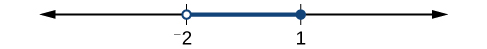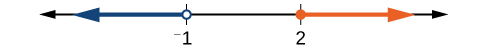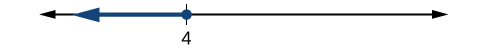## Section Exercises

1. When solving an inequality, explain what happened from Step 1 to Step 2:

$\begin{array}{ll}\text{Step 1}\hfill & -2x>6\hfill \\ \text{Step 2}\hfill & x<-3\hfill \end{array}$

2. When solving an inequality, we arrive at

$\begin{array}{l}x+2< x+3\hfill \\ 2< 3\hfill \end{array}$

Explain what our solution set is.

3. When writing our solution in interval notation, how do we represent all the real numbers?

4. When solving an inequality, we arrive at

$\begin{array}{l}x+2>x+3\hfill \\ 2>3\hfill \end{array}$

Explain what our solution set is.

5. Describe how to graph $y=|x - 3|$

For the following exercises, solve the inequality. Write your final answer in interval notation.

6. $4x - 7\le 9$

7. $3x+2\ge 7x - 1$

8. $-2x+3>x - 5$

9. $4\left(x+3\right)\ge 2x - 1$

10. $-\frac{1}{2}x\le \frac{-5}{4}+\frac{2}{5}x$

11. $-5\left(x - 1\right)+3>3x - 4-4x$

12. $-3\left(2x+1\right)>-2\left(x+4\right)$

13. $\frac{x+3}{8}-\frac{x+5}{5}\ge \frac{3}{10}$

14. $\frac{x - 1}{3}+\frac{x+2}{5}\le \frac{3}{5}$

For the following exercises, solve the inequality involving absolute value. Write your final answer in interval notation.

15. $|x+9|\ge -6$

16. $|2x+3|<7$

17. $|3x - 1|>11$

18. $|2x+1|+1\le 6$

19. $|x - 2|+4\ge 10$

20. $|-2x+7|\le 13$

21. $|x - 7|<-4$

22. $|x - 20|>-1$

23. $|\frac{x - 3}{4}|<2$

For the following exercises, describe all the x-values within or including a distance of the given values.

24. Distance of 5 units from the number 7

25. Distance of 3 units from the number 9

26. Distance of 10 units from the number 4

27. Distance of 11 units from the number 1

28. $-4<3x+2\le 18$

29. $3x+1>2x - 5>x - 7$

30. $3y<5 - 2y<7+y$

31. $2x - 5<-11\text{ or }5x+1\ge 6$

32. $x+7<x+2$

For the following exercises, graph the function. Observe the points of intersection and shade the x-axis representing the solution set to the inequality. Show your graph and write your final answer in interval notation.

33. $|x - 1|>2$

34. $|x+3|\ge 5$

35. $|x+7|\le 4$

36. $|x - 2|<7$

37. $|x - 2|<0$

For the following exercises, graph both straight lines (left-hand side being y1 and right-hand side being y2) on the same axes. Find the point of intersection and solve the inequality by observing where it is true comparing the y-values of the lines.

38. $x+3<3x - 4$

39. $x - 2>2x+1$

40. $x+1>x+4$

41. $\frac{1}{2}x+1>\frac{1}{2}x - 5$

42. $4x+1<\frac{1}{2}x+3$

For the following exercises, write the set in interval notation.

43. $\{x|-1<x<3\}$

44. $\{x|x\ge 7\}$

45. $\{x|x<4\}$

46. $\{x|x\text{ is all real numbers}\}$

For the following exercises, write the interval in set-builder notation.

47. $\left(-\infty ,6\right)$

48. $\left(4,+\infty \right)$

49. $\left[-3,5\right)$

50. $\left[-4,1\right]\cup \left[9,\infty \right)$

For the following exercises, write the set of numbers represented on the number line in interval notation.

51.52.53.For the following exercises, input the left-hand side of the inequality as a Y1 graph in your graphing utility. Enter y2 = the right-hand side. Entering the absolute value of an expression is found in the MATH menu, Num, 1:abs(. Find the points of intersection, recall (2nd CALC 5:intersection, 1st curve, enter, 2nd curve, enter, guess, enter). Copy a sketch of the graph and shade the x-axis for your solution set to the inequality. Write final answers in interval notation.

54. $|x+2|-5< 2$

55. $\frac{-1}{2}|x+2|< 4$

56. $|4x+1|-3> 2$

57. $|x - 4|< 3$

58. $|x+2|\ge 5$

59. Solve $|3x+1|=|2x+3|$

60. Solve ${x}^{2}-x>12$

61. $\frac{x - 5}{x+7}\le 0$, $x\ne -7$

62. $p=-{x}^{2}+130x - 3000$ is a profit formula for a small business. Find the set of x-values that will keep this profit positive.

63. In chemistry the volume for a certain gas is given by $V=20T$, where V is measured in cc and T is temperature in ºC. If the temperature varies between 80ºC and 120ºC, find the set of volume values.

64. A basic cellular package costs $20/mo. for 60 min of calling, with an additional charge of$.30/min beyond that time. The cost formula would be $C=\20+.30\left(x - 60\right)$. If you have to keep your bill lower than \$50, what is the maximum calling minutes you can use?